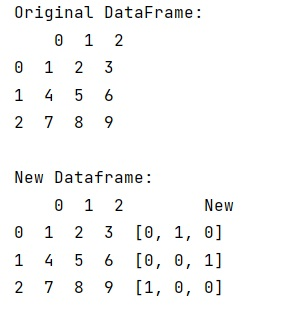# Add NumPy array as column to Pandas dataframe

Learn, how to add NumPy array as column to Pandas dataframe?
Submitted by Pranit Sharma, on January 08, 2023

## Prerequisite

• Python NumPy: NumPy is an abbreviated form of Numerical Python. It is used for different types of scientific operations in python. Numpy is a vast library in python which is used for almost every kind of scientific or mathematical operation. It is itself an array which is a collection of various methods and functions for processing the arrays.
• Python Pandas: Pandas is a special tool that allows us to perform complex manipulations of data effectively and efficiently. Inside pandas, we mostly deal with a dataset in the form of DataFrame. DataFrames are 2-dimensional data structures in pandas. DataFrames consist of rows, columns, and data.

## Problem statement

Given a Pandas DataFrame, you have add/convert a NumPy array as column to the given DataFrame.

## Add NumPy array as column to Pandas dataframe

To add NumPy array as column to Pandas dataframe, you can use toarray() which we will use to convert the NumPy array into an array that will be added to the dataframe as a new column.

Let us understand with the help of an example,

## Example

```# Import numpy
import numpy as np

# Import pandas
import pandas as pd

# Import sparse
import scipy.sparse as sparse

# Creating a dataframe
df = pd.DataFrame(np.arange(1,10).reshape(3,3))

# Display original dataframe
print("Original DataFrame:\n",df,"\n")

# Creating a matrix and then adding it as a column in df
arr = sparse.coo_matrix(([1,1,1], ([0,1,2], [1,2,0])), shape=(3,3))
df['New'] = arr.toarray().tolist()

# Display new df
print("New Dataframe:\n",df,"\n")
```

## Output

The output of the above program will be: